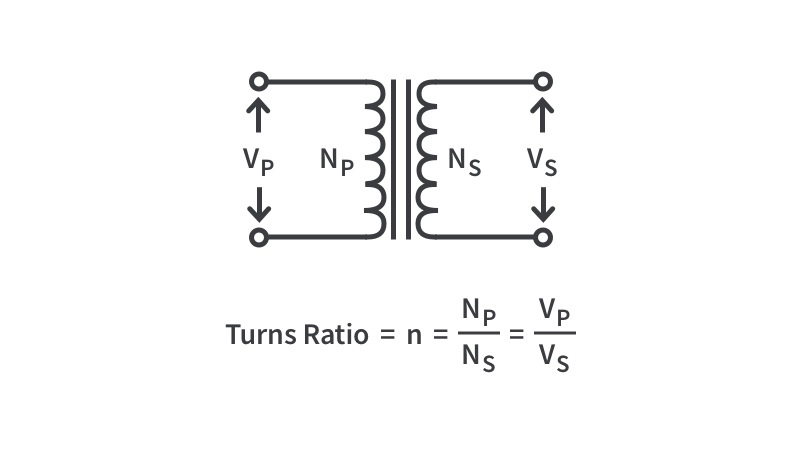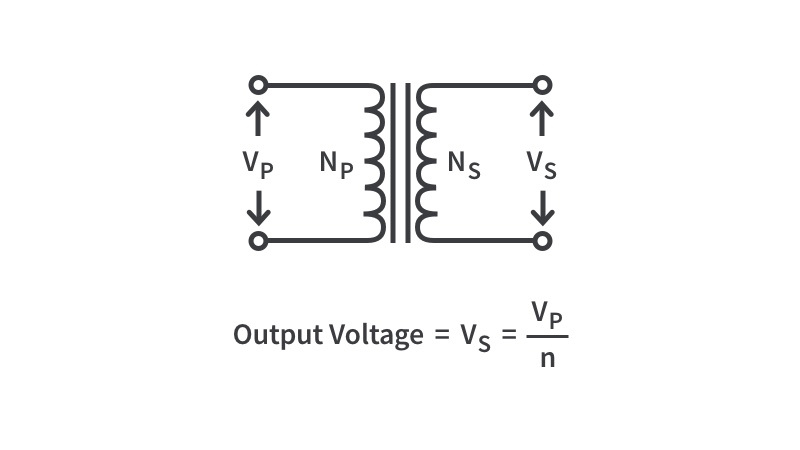# Transformer TurnsInput

– Or –Output
:

One of the beautiful things about AC is how easy it is to change voltages. With a simple ratio between the amount of turns on the primary versus the secondary coil of a transformer, you can easily figure out the voltage in versus the voltage out as well as the current in and out. If you know the ratio and the input, you can find the output. If you know the ratio and the output, you can find the input. If you don’t know the ratio but know 3 out of the 4 variables of voltage input/output and current input/output, then we can figure out the last variable as well.Input | Turns Ratio
:Output
V